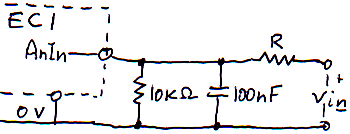NOTICE: SPLat Controls has moved. We are now at 1/85 Brunel Rd, Seaford, 3198. map

In this EasyStep you get a quick overview of the standard analog inputs on the EC1

Expert summary

• Input range 0 to 3.3V using processor's 3.3V supply as the reference;
• fAnIn instruction gives a normalised reading 0.0 to 1.0 in floating point register W
• Accuracy: ~2.5%
• Resolution: 12 bits;
• Maximum signal source impedance: 10KΩ. Suggest 100nF ceramic across the input;
• Inputs outside 0-3.3V can upset adjacent channels or damage the chip.

Close

An analog input is a pin that measures the voltage level of something connected to it. Whereas a digital input can only distinguish high/low voltages, an analog input returns a number that is a direct measure of the magnitude of the input voltage. This makes it useful for measuring continuously variable quantities such as (with a suitable sensor) temperature or the position of a potentiometer in a joystick.

• The voltage applied to the pin should be between 0V and the board's 3.3V internal supply voltage. Small excursions (<300mV) beyond these limits may introduce errors in other analog input readings. Large excursions can damage the board;
• The output resistance of the signal source should be as low as possible. More than 10KΩ will introduce errors;
• It is a good idea to use a 10nF ceramic capacitor between the analog input pin and 0V.

When your program executes an fAnIn 0 instruction, the voltage on analog input 0 is measured, and returned as a number between 0.0 and 1.0 in the floating point register W. 0.0 corresponds to 0V in, 1.0 corresponds to 3.3V (the reference voltage).

## Resolution

The analog inputs have 12-bit resolution. That means the input voltage range is divided into 2^12 (4096) steps. This corresponds to 0.806mV or 0.025% of full scale. To put this in context: With a typical thermistor temperature measurement setup it can resolve changes as low as 0.05°C (0.1°F). If you could feed it from a 1m (3') long potentiometer, it could detect movements of 0.25mm (0.01")

## Accuracy

While the resolution is 0.025%, the accuracy is nowhere near that. See Analog Accuracy is expensive, Resolution is cheap The main governing factor of accuracy of any analog to digital converter is the accuracy of the reference voltage. In the EC1 the reference voltage is the 3.3V supply, which comes from a MCP1801 3.3V regulator with a worst case accuracy of 2.5% (it is typically better than that, but professionals use worst case numbers!). If you have a good digital multimeter, you can get a "fix" on the actual reference voltage by measuring between a 0V pin and a 3.3V pin.

If you need more accuracy you will have to somehow calibrate your system.

## Scaling for input voltage ranges greater than 3.3V nominal

You can use a simple voltage divider to divide down the voltage into the analog input. Here's a basic circuit:I've set the "bottom" resistor at a fixed 10KΩ, and shown a recommended 100nF ceramic capacitor for noise reduction. The value of the other resistor 'R' depends on the full scale voltage range you want to measure.

Click here for a table of values of R for different input voltage ranges.

The values given assume a worst case low reference voltage, which will ensure that the given voltage range will always fall within that range of the EC1's analog to digital converter. In practice that means the full scale voltage given will typically give a normalised reading of 0.975 rather than 1.0.

 R Voltage range 1KΩ 3.539 1100Ω 3.571 1200Ω 3.604 1300Ω 3.636 1500Ω 3.700 1600Ω 3.732 1800Ω 3.797 2KΩ 3.861 2200Ω 3.925 2400Ω 3.990 2700Ω 4.086 3KΩ 4.183 3300Ω 4.279 3600Ω 4.376 3900Ω 4.472 4300Ω 4.601 4700Ω 4.730 5100Ω 4.858 5600Ω 5.019 6200Ω 5.212 6800Ω 5.405 7500Ω 5.631 8200Ω 5.856 9100Ω 6.145 10KΩ 6.435 11KΩ 6.757 12KΩ 7.079 13KΩ 7.400 15KΩ 8.044 16KΩ 8.366 18KΩ 9.009 20KΩ 9.653 22KΩ 10.296 24KΩ 10.940 27KΩ 11.905 30KΩ 12.870 33KΩ 13.835 36KΩ 14.801 39KΩ 15.766 43KΩ 17.053 47KΩ 18.340 51KΩ 19.627 56KΩ 21.236 62KΩ 23.166 68KΩ 25.097 75KΩ 27.349 82KΩ 29.601 91KΩ 32.497

Close

## Some interesting ways to blow up your EC1

If you want to blow up your EC1, and void the warranty, here are some things you can do to the analog inputs: